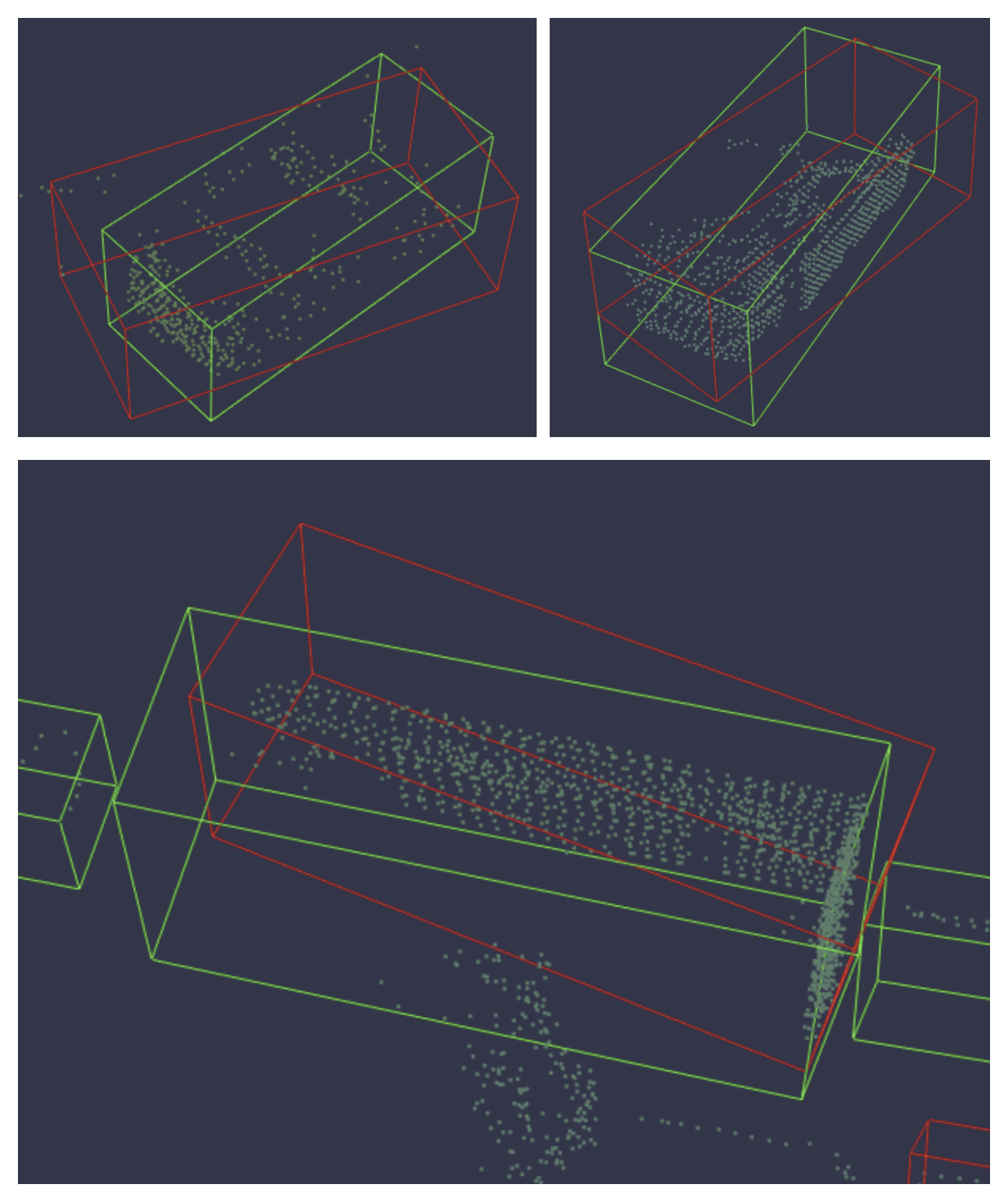# Introduction

In this report, I'll show you how to visualize a model's predictions with Weights & Biases – images, videos, audio, tables, HTML, metrics, plots, 3d objects and point clouds.

## Try it in this Kaggle kernel →

If you have any questions, we'd love to answer them.

# Metrics

Calling `wandb.log(dict)` logs the keys and values of the dictionary passed in and associates the values with a step.

### Example

``````# Initialize run
wandb.init()

# Log multiple metrics
wandb.log({'accuracy': 0.9, 'epoch': 5})

# Log metrics in a loop
for price in apple['close']:
wandb.log({"Stock Price": price})
``````

# Plots

You can pass a matplotlib pyplot or figure object into `wandb.log`.

By default we'll convert the plot into a plotly plot. If you want to explictly log the plot as an image, you can pass the plot into wandb.Image. We also accept directly logging plotly charts.

### Example

``````import matplotlib.pyplot as plt
fibonacci = [0, 1, 1, 2, 3, 5, 8, 13, 21, 34]
plt.plot(fibonacci)
plt.ylabel('some interesting numbers')

# Initialize run
wandb.init()

# Log plot object
wandb.log({"plot": plt})
``````

# Images

If the image is a numpy array, we assume it's gray scale if the last dimension is 1, RGB if it's 3, and RGBA if it's 4.

If the array contains floats we convert them to ints between 0 and 255. You can specify a mode manually or just supply a PIL.Image. It's recommended to log fewer than 50 images per step. Click "Create Visualization" to customize the image gallery.

### Example

``````from matplotlib import pyplot as plt
path_to_img = "../input/kernel-files/cafe.jpg"

# Initialize run
wandb.init()

# Log image(s)
wandb.log({"img": [wandb.Image(im, caption="Cafe")]})
``````

# Videos

If the video is a numpy array, we assume the dimensions are: time,channels,width,height. By default we create a 4 fps gif image (ffmpeg and the moviepy python library is required when passing numpy objects).

Supported formats are "gif", "mp4", "webm", and "ogg". If you pass a string to wandb.Video we assert the file exists and is a supported format before uploading to wandb. Passing a BytesIO object will create a tempfile with the specified format as the extension. On the W&B runs page, you will see your videos in the Media section.

### Example

``````path_to_video = "../input/kernel-files/openai-gym.mp4"

# Initialize run
wandb.init()

# Log video(s)
wandb.log({"video": wandb.Video(path_to_video, fps=4, format="gif")})
``````

# Audio

The maximum number of audio clips that can be logged per step is 100.

### Example

``````from scipy.io import wavfile
path_to_audio = "../input/kernel-files/piano.wav"

# Initialize run
wandb.init()

# Log audio file
wandb.log({"examples": [wandb.Audio(path_to_audio, caption="Piano", sample_rate=32)]})
``````

# 3D Objects

We support logging 3D file types of in three different formats: `glTF, glb, obj`. The 3D files will be viewable on the run page upon completion of your run.

### Example

``````path_to_obj = "../input/kernel-files/wolf.obj"

# Initialize run
wandb.init()

# Log 3D object
wandb.log({"3d_object": wandb.Object3D(open(path_to_obj))})
``````

# Point Clouds

Point Clouds logging has currently has two modes.

Logging a single set of points representing an object , useful for representing datasets like ShapeNet(Example Report).

Along with a new beta release lidar scene renderer. Logging a set of points is as simple as passing in a numpy array containing your coordinates and the desired colors for the points.

Three different shapes of numpy arrays are supported for flexible color schemes, supporting common ML us

• `[[x, y, z], ...]` nx3
• `[[x, y, z, c], ...] nx4 | c` is a category in the range [1, 14] (Useful for segmentation)
• `[[x, y, z, r, g, b], ...] nx6 | r,g,b` are values in the range [0,255]for red, green, and blue color channels.

### Example

``````# Initialize run
wandb.init()

# Log points and boxes
wandb.log(
{
"point_scene": wandb.Object3D(
{
"type": "lidar/beta",
"points": np.array(
[
[0.4, 1, 1.3],
[1, 1, 1],
[1.2, 1, 1.2]
]
),
"boxes": np.array(
[
{
"corners": [
[0,0,0],
[0,1,0],
[0,0,1],
[1,0,0],
[1,1,0],
[0,1,1],
[1,0,1],
[1,1,1]
],
"label": "Box",
"color": [123,321,111],
},
{
"corners": [
[0,0,0],
[0,2,0],
[0,0,2],
[2,0,0],
[2,2,0],
[0,2,2],
[2,0,2],
[2,2,2]
],
"label": "Box-2",
"color": [111,321,0],
}
]
),
}
)
}
)
``````# HTML

Custom html can be logged at any key, this exposes an HTML panel on the run page. By default we inject default styles, you can disable default styles by passing `inject=False`.

### Example

``````path_to_html = "../input/kernel-files/some_html.html"

# Initialize run
wandb.init()

# Log plot HTML
# As a file
wandb.log({"custom_file": wandb.Html(open(path_to_html))})

# Inline

# Disable default styles
wandb.log({"custom_file": wandb.Html(open("some.html"), inject=False)})
``````

# Incremental Logging

If you want to log to a single history step from lots of different places in your code you can pass a step index to wandb.log() as shown in the example below.

wandb.log(dict) accepts a few keyword arguments:

• `step` — Step to associate the log with (see Incremental Logging)
• `commit` — If true, increments the step associated with the log(default: true)

### Example

``````# Initialize run
wandb.init()

# Log the metric to specific steps
for step, price in enumerate(apple['close']):
# Specify steps
wandb.log({"Stock Price": price}, step=step)

# Initialize a new run
wandb.init(project="visualize-metrics", name="incremental_logging")

# Log many metrics to the same step
for close, date in zip(apple['close'], apple['date']):
# Log multiple metrics to the same step
wandb.log({"Stock Price": close}, commit=False)

# Somewhere else when I'm ready to report this step:
wandb.log({'Another Metric': close*10})
``````

As long as you keep passing the same value for `step`, W&B will collect the keys and values from each call in one unified dictionary. As soon you call `wandb.log()` with a different value for `step` than the previous one, W&B will write all the collected keys and values to the history, and start collection over again.

Note that this means you should only use this with consecutive values for `step`: 0, 1, 2, .... This feature doesn't let you write to absolutely any history step that you'd like, only the "current" one and the "next" one.

You can also set `commit=False` in `wandb.log` to accumulate metrics, just be sure to call `wandb.log` without the `commit` flag to persist the metrics.